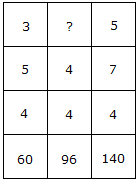# Verbal Reasoning - Character Puzzles - Discussion

### Discussion :: Character Puzzles - Character Puzzles 1 (Q.No.39)

39.

Which one will replace the question mark ?[A]. 4 [B]. 6 [C]. 9 [D]. 8

Explanation:

3 x 5 x 4 = 60

and 5 x 7 x 4 = 140

Therefore, 4 x 4 x ? = 96

? = (96/16) = 6.